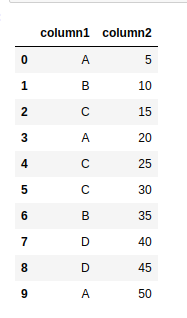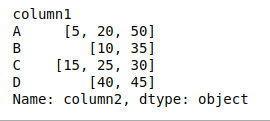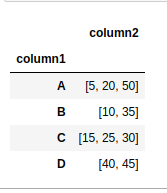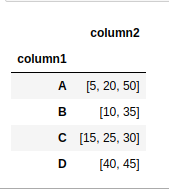# How to group dataframe rows into list in Pandas Groupby?

• Last Updated : 02 Feb, 2021

Suppose you have a pandas DataFrame consisting of 2 columns and we want to group these columns. In this article, we will discuss about the same. First, let;s create the dataframe.

## Python3

 `# importing pandas as pd``import` `pandas as pd`` ` ` ` `# Create the data frame``df ``=` `pd.DataFrame({``'column1'``: [``'A'``, ``'B'``, ``'C'``, ``'A'``, ``'C'``,``                               ``'C'``, ``'B'``, ``'D'``, ``'D'``, ``'A'``],``                   ``'column2'``: [``5``, ``10``, ``15``, ``20``, ``25``, ``30``, ``                             ``35``, ``40``, ``45``, ``50``]})`` ` `# Print the dataframe``df`

Output:Example #1: We can use groupby() method on column 1 and apply the method to apply a list on every group of pandas DataFrame.

## Python3

 `# importing pandas as pd``import` `pandas as pd`` ` ` ` `# Create the data frame``df ``=` `pd.DataFrame({``'column1'``: [``'A'``, ``'B'``, ``'C'``, ``'A'``, ``'C'``,``                               ``'C'``, ``'B'``, ``'D'``, ``'D'``, ``'A'``],``                   ``'column2'``: [``5``, ``10``, ``15``, ``20``, ``25``, ``30``, ``                             ``35``, ``40``, ``45``, ``50``]})`` ` ` ` `# Use groupby method and apply``# method on the dataframe``df ``=` `df.groupby(``'column1'``)[``'column2'``].``apply``(``list``)`` ` `# Print the dataframe again``df`

Output:Example #2: We can use groupby() method on column 1 and agg() method to apply aggregation, consisting of the lambda function, on every group of pandas DataFrame.

## Python3

 `# importing pandas as pd``import` `pandas as pd`` ` ` ` `# Create the dataframe``df ``=` `pd.DataFrame({``'column1'``: [``'A'``, ``'B'``, ``'C'``, ``'A'``, ``'C'``, ``                               ``'C'``, ``'B'``, ``'D'``, ``'D'``, ``'A'``], ``                   ``'column2'``: [``5``, ``10``, ``15``, ``20``, ``25``, ``30``,``                               ``35``, ``40``, ``45``, ``50``]})`` ` `# Use groupby method and agg method ``# with lambda function on the dataframe``df ``=` `df.groupby(``'column1'``).agg({``'column2'``: ``lambda` `x: ``list``(x)})`` ` `# Print the dataframe again``df`

Output:Example #3: We can use the groupby() method on column 1 and agg() method to apply the aggregation list, on every group of pandas DataFrame.

## Python3

 `# importing pandas as pd``import` `pandas as pd`` ` ` ` `# Create the data frame``df ``=` `pd.DataFrame({``'column1'``: [``'A'``, ``'B'``, ``'C'``, ``'A'``, ``'C'``,``                               ``'C'``, ``'B'``, ``'D'``, ``'D'``, ``'A'``],``                   ``'column2'``: [``5``, ``10``, ``15``, ``20``, ``25``, ``30``, ``                               ``35``, ``40``, ``45``, ``50``]})`` ` `# Use groupby method and agg method ``# with list as argument on the dataframe``df ``=` `df.groupby(``'column1'``).agg(``list``)`` ` `df`

Output:Example #4: We can use groupby() method on column 1 and agg() method by passing ‘pd.Series.tolist’ as an argument.

## Python3

 `# importing pandas as pd``import` `pandas as pd`` ` ` ` `# Create the data frame``df ``=` `pd.DataFrame({``'column1'``: [``'A'``, ``'B'``, ``'C'``, ``'A'``, ``'C'``, ``                               ``'C'``, ``'B'``, ``'D'``, ``'D'``, ``'A'``], ``                   ``'column2'``: [``5``, ``10``, ``15``, ``20``, ``25``, ``30``,``                               ``35``, ``40``, ``45``, ``50``]})`` ` `# Use groupby method and agg method with``# pd.Series.tolist as argument on the dataframe``df ``=` `df.groupby(``'column1'``).agg(pd.Series.tolist)`` ` `df`

Output:My Personal Notes arrow_drop_up